# CrystalExplorer Model Energies

The energy of interaction between molecules is commonly expressed in terms of four key components: electrostatic, polarization, dispersion, and exchange-repulsion:

$E_{\text{tot}} = k_{\text{ele}} E_{\text{ele}} + k_{\text{pol}} E_{\text{pol}} + k_{\text{dis}} E_{\text{dis}} + k_{\text{rep}} E_{\text{rep}}$

Using monomer wavefunctions at HF/3-21G, MP2/6-31G(d,p) and B3LYP/6-31G(d,p) levels to obtain accurate values of electrostatic, polarization and repulsion energies, along with Grimme’s D2 dispersion corrections, we originally derived three energy models by fitting to dispersion-corrected DFT energies for a large number of pairs of neutral molecules extracted from organic (and a few inorganic) molecular crystals. The fitting process involved modifying the scale factors in the equation above to obtain the lowest MAD between model energies and those in the training set.

The best performing model in that initial work reproduced B3LYP-D2/6-31G(d,p) counterpoise-corrected energies with a mean absolute deviation (MAD) of just over 1 kJ/mol but in considerably less computation time. It also performed surprisingly well against benchmark CCSD(T)/CBS energies, with a MAD of 2.5 kJ/mol for a combined data set including Hobza’s X40, S22, A24, and S66 dimers.

We have recently expanded the realm of application of the two preferred energy models - CE-HF and CE-B3LYP - to include metal coordination compounds, organic salts and solvates, as well as open shell molecules (radicals). This involved calculation of energies for molecule/ion pairs extracted from 171 crystal structures. The mean absolute deviation of CE-B3LYP model energies from DFT values is a modest 2.4 kJ/mol for pairwise energies that span a range of 3.75 MJ/mol. For the less accurate - but faster - CE-HF model the MAD is 4.7 kJ/mol. These two energy models have found widespread application in investigations of molecular crystals.

## Interaction Energy for a Pair of Molecules​

The simplest approach is to select a pair of molecules in the graphics window, then click on the Calculate Energies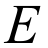button in the toolbar. This will bring up the Calculate Interaction Energy dialog.

Here the choice can be made between the CE-B3LYP and CE-HF energy models, or energies can be computed using wavefunctions defined by the user (e.g., MP2; different basis set). There are also advanced options to edit input files if necessary.

danger

Although other levels of theory and/or basis sets can be chosen, the model energies are only calibrated for CE-B3LYP and CE-HF models. In other words, CrystalExplorer only includes optimum scale factors for these models.

## Interaction Energies for a Cluster​

### Only one unique molecue in the unit cell - $Z' = 1$​

Perhaps the best (and most efficient) way to explore the energies of interaction between molecules in your crystal structure is to perform energy calculations for a cluster of molecules. This means the molecular wavefunction is computed once only; symmetry operations are used to generate wavefunctions and electron densities for all symmetry-related molecules.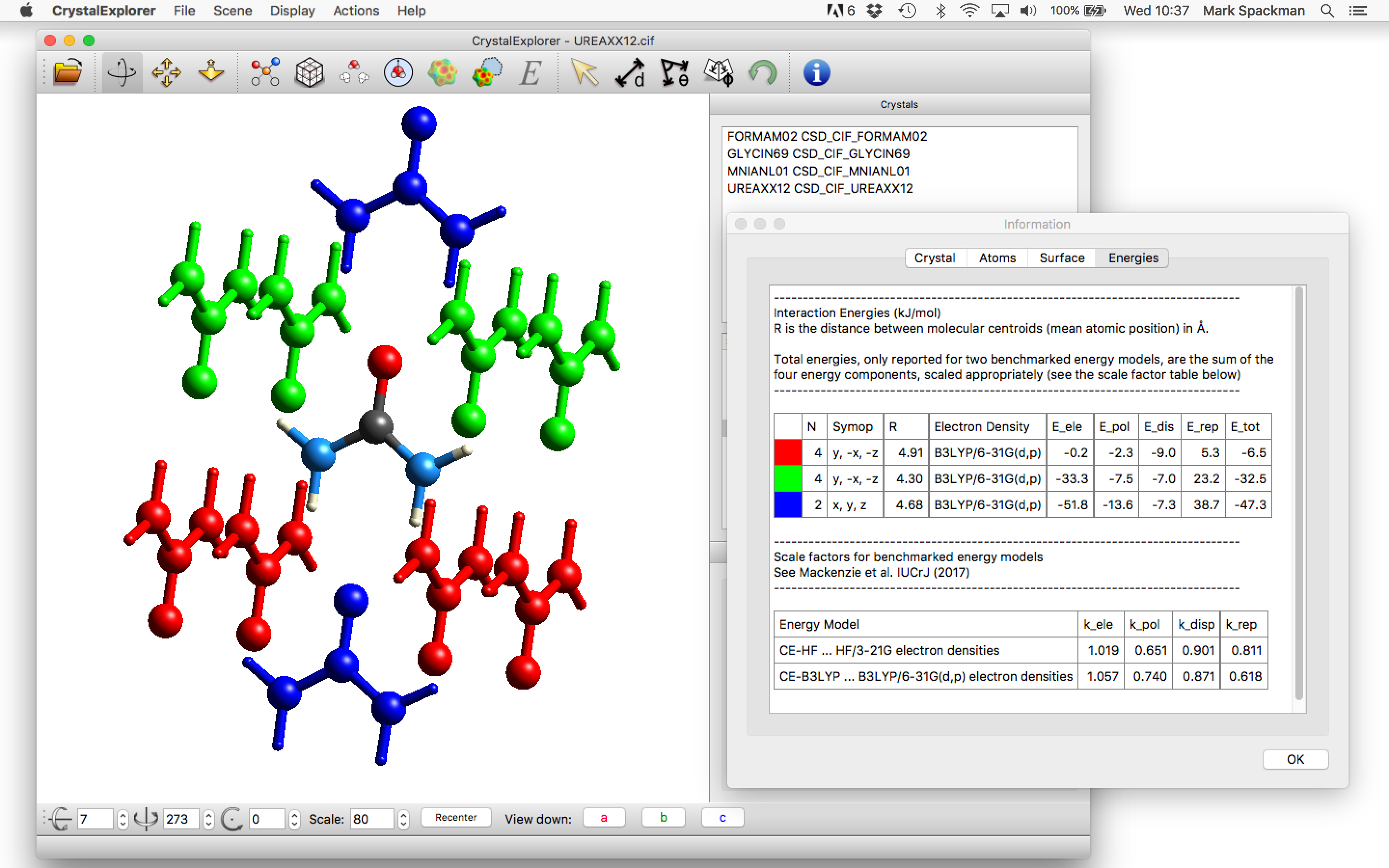To do this first select the molecule and click on the Calculate Energiesbutton in the toolbar. This will first bring up a dialog asking if you wish to generate energies for a 3.8 Å cluster of molecules around the selected molecule. After clicking Yes, the desired cluster will be created and the Calculate Interaction Energy dialog will appear as above.

Once the choice of energy models is made, click OK and the wavefunction will be computed, after which all interaction energies between the selected molecule (at the centre of the cluster) and its neighbours will be computed. Upon completion of the energy calculations the molecules in the graphics window (see below, left) will be colour coded to uniquely identify them with the energy (and energy components) in the Information dialog: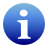### More than one unique molecule in the unit cell​

info

It is highly recommended, in order to work around some bugs in the current logic of the program, to manually calculate some one pair interaction between each symmetry unique molecule before following the rest of this procedure. The easiest way to do this is to select select a pair of molecules (one of each kind) and click the calculate energies button - which will calculate the energy just for that pair.

CrystalExplorer will calculate interaction energies for all pairs of molecules $AB$ where $A$ is the currently selected molecule and each $B$ is another molecule currently displayed. As such, in order to calculate interactions for clusters surrounding each molecule, we suggest the following procedure for each unique molecule (or ion, fragment etc.) in the crystal:

1. Select (highlight) the molecule $A$.
2. Click the Generate Atoms Within Radius button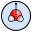with the desired radius (default is 3.80 Å).
3. Click the Complete Fragments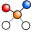button in order to complete all molecular fragments.
4. Click the Calculate Energiesbutton.

This will calculate all energies of interactions between neighbouring molecules currently displayed and the central selected molecule. Once this is performed for each individual molecular fragment, the information tables should be populated with the desired interactions.

## Some Comments on the Information Dialog​

The table of energies provided by this dialog provides the following for each interaction energy (row):

• A colour to assist in the identification of the interaction energy with a particular molecule of that colour and the central molecule in the graphics window;
• The number of pairs, $N$, in the graphics window with that energy and other characteristics (helpful for brute force calculation of lattice energies);
• The symop relating that particular colour coded molecule with the central molecule. Only the rotation is given, and in the example of oxalic acid dihydrate above it can be seen that there is (quite sensibly) no symop relating the oxalic acid and water molecules;
• The distance (Å) between molecular centroids for the particular pair. The centroids are based only on the coordinates of all atoms, o they are not centres of mass;
• The electron density model used in the calculation;
• The individual components ($E_{ele}$, $E_{pol}$, $E_{dis}$, $E_{rep}$) and total energy, $E_{tot}$, in kJ/mol. Important: The total energy is the sum of scaled components (using the scale factors appropriate to the model, as given at the bottom of the dialog), but the separate components are not scaled.
• For crystals with more than one molecule/ion in the asymmetric unit ($Z' > 1$) the table of energies will contain duplicates. This is a known problem with the implementation of energies in CrystalExplorer, but it only causes some inconvenience. It does not compromise the energy framework diagrams, but care will obviously be needed if energies are summed to estimate a lattice energy.

## Using Tonto instead of Gaussian wavefunctions​

If you don't have a Gaussian license - our suggestion is to use NWChem with CrystalExplorer21 or newer.

danger

THIS OPTION IS UNDER DEVELOPMENT AND MAY STILL GIVE ERRORS IN SOME CASES. USE WITH CAUTION AND CRITCALLY ASSESS ALL ENERGIES!

All of the calibration of CE-HF and CE-B3LYP model energies has been performed using molecular electron densities derived from wavefunctions computed by Gaussian09. However Tonto, which is a backend to CrystalExplorer, can also be used to compute ab initio and DFT wavefunctions. CE-HF model energies produced using Tonto HF/3-21G electron densities are identical with those obtained using Gaussian electron densities. At present CE-B3LYP model energies based on Tonto B3LYP/6-31G(d,p) electron densities differ slightly from those obtained using Gaussian electron densities, because the Tonto implementation of B3LYP doesn't use an identical integration grid to that in Gaussian. The difference in CE-B3LYP model energies is typically ~0.2 kJ/mol, but can be as much as 2 kJ/mol.

In summary, if you do not have a copy of Gaussian then identical CE-HF model energies can be obtained using Tonto to compute the HF/3-21G wavefunctions. This is done by clicking Energies from user-defined wavefunction in the Calculate Interaction Energy dialog, where Tonto can be chosen instead of Gaussian. CE-B3LYP model energies can also be obtained this way, but at present they differ slightly from those that will be obtained with Gaussian B3LYP/6-31G(d,p) wavefunctions. However, energy frameworks produced from Tonto-derived model energies will show no visible difference.

danger

There is presently a bug when using Tonto wavefunctions to compute energies for co-crystals, where the semi-automatic procedure above will result in NaN and wrong energies. To avoid this you need to make sure that Tonto wavefunctions exist for all unique molecules before going ahead and computing energies for all pairs in each cluster. To do this you could compute Hirshfeld surfaces mapped with electron density for all unique molecules (using the wavefunction you actually want for energies of course). Or you could first compute energies for pairs of molecules making sure they include all unique molecules. Both of these processes will guarantee that the Tonto wavefunctions you need are computed and stored so that they can be re-used for any dimer energy calculation.